# Multiplication - 5th grade (10y) - math problems

#### Number of problems found: 107

• The largest numberFind the largest integer such that: 1. No figures is not repeat, 2. multiplication of every two digits is odd, 3. addition all digits is odd.
• Hours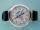How many hours is 9 days?
• Written numberPlace+values x ten thousands =30 thousands
• MilimetersHow many millimeters are 1/4 meters?
• Weeks38 weeks is equal to how many days?
• Seeds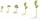Of the 72 wet seeds, 7/8 germinated. How many seeds germinate?
• How manzBy how many are the product of the numbers 328 and 7 greater than their sum?
• Expression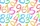If A=2 B=3 evaluate expression A(B+A) and multiply it by A
• Numbers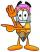By how many is the difference of numbers 8 and 34 less than its product?
• Women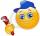In a company of 1050 employees are 2/3 women. How many women work in the company?
• About whatHow much and how many times is 72.1 greater than 0.00721?
• Operations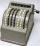Sum of the numbers 1.01 and 3.35 multiply by the difference of numbers 6.69 and 1.39.
• Unknown number 2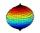I think the number. When he reduces it four times, I'll get 11. What number am I thinking?
• Evaluate expressionIf x=2, y=-5 and z=3 what is the value of x-2y
• Products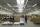15 products are 48 € more expensive than 12 products. How many euros will it cost five same products?
• Computer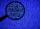A print line on a computer contains 64 characters (letters, spacers, or other chars). Find how many characters there are in 7 lines.
• Hexagon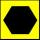Draw a regular hexagon inscribed in a circle with radius r=8 cm. What is its perimeter?
• ProductResult of the product of the numbers 1, 2, 3, 1, 2, 0 is:
• Wallpaper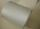Salesman account for 2.3 meters of wallpaper 149.50 Kc. How much cost 1 meter of wallpaper?
• Expression 6Evaluate expression: -6-2(4-8)-9

Do you have an interesting mathematical word problem that you can't solve it? Submit a math problem, and we can try to solve it.

We will send a solution to your e-mail address. Solved examples are also published here. Please enter the e-mail correctly and check whether you don't have a full mailbox.

Please do not submit problems from current active competitions such as Mathematical Olympiad, correspondence seminars etc...

Multiplication Problems. Examples for 5th grade (the fifth graders).